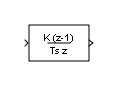# Discrete Derivative

Compute discrete-time derivative

• Library:

•## Description

The Discrete Derivative block computes an optionally scaled discrete time derivative as follows

`$y\left({t}_{n}\right)=K\left(\frac{u\left({t}_{n}\right)-u\left({t}_{n-1}\right)}{{T}_{s}}\right)$`

where

• $u\left({t}_{n}\right)$ and $y\left({t}_{n}\right)$ are the block input and output at the current time step, respectively.

• $u\left({t}_{n-1}\right)$ is the block input at the previous time step.

• $K$ is an optional scaling factor, specified using the Gain value parameter.

• ${T}_{s}$ is the simulation's discrete step size, which must be fixed.

Note

Do not use this block in subsystems with a nonperiodic trigger (for example, nonperiodic function-call subsystems). This configuration produces inaccurate results.

## Ports

### Input

expand all

Input signal, specified as a scalar, vector, or matrix.

Data Types: `single` | `double` | `int8` | `int16` | `int32` | `int64` | `uint8` | `uint16` | `uint32` | `uint64` | `fixed point`

### Output

expand all

Optionally scaled discrete-time derivative, specified as a scalar, vector, or matrix. For more information on how the block computes the discrete-time derivative, see Description. You specify the data type of the output signal with the Output data type parameter.

Data Types: `single` | `double` | `int8` | `int16` | `int32` | `int64` | `uint8` | `uint16` | `uint32` | `uint64` | `fixed point`

## Parameters

expand all

### Main

Scaling factor applied to the computed derivative, specified as a real scalar value.

#### Programmatic Use

 Block Parameter: `gainval` Type: character vector Values: scalar Default: `'1.0'`

Initial condition for the previous scaled input, specified as a scalar.

#### Programmatic Use

 Block Parameter: `ICPrevScaledInput` Type: character vector Values: scalar Default: `'0.0'`

Specify whether the block performs sample- or frame-based processing:

• `Columns as channels (frame based)` — Treat each column of the input as a separate channel (frame-based processing).

Note

Frame-based processing requires a DSP System Toolbox™ license.

• `Elements as channels (sample based)` — Treat each element of the input as a separate channel (sample-based processing).

Use Input processing to specify whether the block performs sample- or frame-based processing. The block accepts frame-based signals for the input `u`. All other input signals must be sample-based.

Input Signal uInput Processing ModeBlock Works?
Sample basedSample basedYes
Frame basedNo, produces an error
Sample basedFrame basedYes
Frame basedYes

#### Programmatic Use

 Block Parameter: `InputProcessing` Type: character vector Values: ```'Columns as channels (frame based)'``` | ```'Elements as channels (sample based)'``` Default: ```'Elements as channels (sample based)'```

### Signal Attributes

Lower value of the output range that Simulink® checks.

Simulink uses the minimum to perform:

Note

Output minimum does not saturate or clip the actual output signal. Use the Saturation block instead.

#### Programmatic Use

 Block Parameter: `OutMin` Type: character vector Values: `'[ ]'`| scalar Default: `'[ ]'`

Upper value of the output range that Simulink checks.

Simulink uses the maximum value to perform:

Note

Output maximum does not saturate or clip the actual output signal. Use the Saturation block instead.

#### Programmatic Use

 Block Parameter: `OutMax` Type: character vector Values: `'[ ]'`| scalar Default: `'[ ]'`

Specify the output data type. You can set it to:

• A rule that inherits a data type, for example, ```Inherit: Inherit via back propagation```

• The name of a built-in data type, for example, `single`

• The name of a data type object, for example, a `Simulink.NumericType` object

• An expression that evaluates to a data type, for example, `fixdt(1,16,0)`

Click the buttonto display the Data Type Assistant, which helps you set the data type attributes. For more information, see Specify Data Types Using Data Type Assistant.

#### Programmatic Use

 Block Parameter: `OutDataTypeStr` Type: character vector Values: ```'Inherit: Inherit via internal rule' | 'Inherit: Inherit via back propagation' | 'double' | 'single' | 'int8' | 'uint8' | 'int16' | 'uint16' | 'int32' | 'uint32' | 'int64' | 'uint64' | 'fixdt(1,16,0)' | 'fixdt(1,16,2^0,0)'``` Default: `'Inherit: Inherit via internal rule'`

Select this parameter to prevent the fixed-point tools from overriding the Output data type you specify on the block. For more information, see Use Lock Output Data Type Setting (Fixed-Point Designer).

#### Programmatic Use

 Block Parameter: `LockScale` Type: character vector Values: `'off' | 'on'` Default: `'off'`

Specify the rounding mode for fixed-point operations. For more information, see Rounding (Fixed-Point Designer).

Block parameters always round to the nearest representable value. To control the rounding of a block parameter, enter an expression using a MATLAB® rounding function into the mask field.

#### Programmatic Use

 Block Parameter: `RndMeth` Type: character vector Values: ```'Ceiling' | 'Convergent' | 'Floor' | 'Nearest' | 'Round' | 'Simplest' | 'Zero'``` Default: `'Floor'`

When you select this check box, overflows saturate to the maximum or minimum value that the data type can represent. Otherwise, overflows wrap.

When you select this check box, saturation applies to every internal operation on the block, not just the output or result. In general, the code generation process can detect when overflow is not possible. In this case, the code generator does not produce saturation code.

#### Programmatic Use

 Block Parameter: `DoSatur` Type: character vector Values: `'off' | 'on'` Default: `'off'`

## Block Characteristics

 Data Types `double` | `fixed point` | `integer` | `single` Direct Feedthrough `no` Multidimensional Signals `no` Variable-Size Signals `yes` Zero-Crossing Detection `no`